# Elasticity

Updated July 2, 2021

## What Is Elasticity?

Elasticity is a measure of the change in one variable in response to a change in another, and it’s usually expressed as a ratio or percentage. In economics, elasticity generally refers to variables such as supply, demand, income, and price. The responsiveness to these changes helps identify and analyze relationships between variables.

### How Is Elasticity Used?

Elasticity has a wide variety of applications in both economics and finance:

Policymakers: Analyze the impact that policies involving taxation, minimum wage, subsidies, etc. have on consumer behavior.
Businesses: Determine the effects a change in price may have on revenue.
Analysts: Measure trends such as the effects a change in income could have on consumer behavior.
Unions: If labor is a major input of a good, unions can use the elasticity of demand of that good to negotiate wages.
Entrepreneurs: Determine if a market is worth entering or if a product is worth selling.

## What Are Elastic Goods?

The elasticity of goods measures sensitivity to price changes. Given a percentage change in price, an elastic good will have a greater percentage change in quantity supplied or demanded.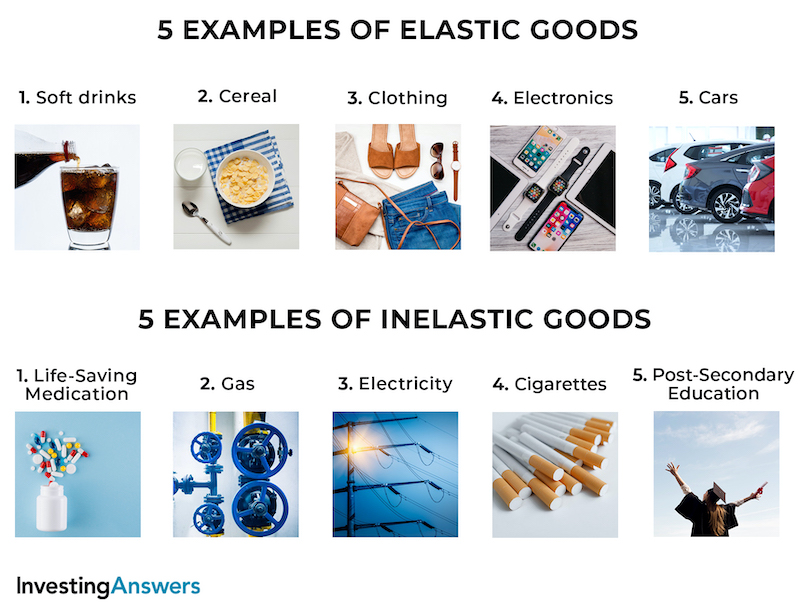Elastic goods are goods that have a significant change in demand or supply in response to a change in price. Generally, these are goods that are not considered necessities, or goods for which there are substitutes readily available.

Using demand as an example, if the price of a good were to decrease by X amount, there would be a greater increase in the amount that people would want to buy. If the price were to increase by X amount, there would be a greater decrease in the amount that people would want to buy.

## 5 Examples of Elastic Goods

Common elastic items include:

### 1. Soft Drinks

Soft drinks aren't a necessity, so a big increase in price would cause people to stop buying them or look for other brands. A big decrease in price, however, would be sure to attract more buyers.

### 2. Cereal

Like soft drinks, cereal isn't a necessity and there are plenty of different choices. A change in prices would have a big impact on the demand.

### 3. Clothing

Similar to the above two examples, any particular item of clothing is not a necessity. Since buyers have a massive number of options, demand is largely affected by price.

### 4. Electronics

Most electronics have multiple options at varying price points. Adjustments to price will greatly influence the demand for an item.

### 5. Cars

While many people shop for specific cars, there are many makes and models. If the price of a car increases, people will consider similar models. On the other hand, if the price decreases, more people will be interested in buying that car.

## What Are Inelastic Goods?

An inelastic good will have a smaller percentage change in quantity demanded/supplied. This indicates that elastic items are more sensitive to changes in price while inelastic items are less sensitive.

## Examples of Inelastic Goods

Inelastic goods don't have a significant change in demand or supply in response to a price change. In general, these are goods that are considered necessary or without many (or any) substitutes.

Using demand as an example, if the price of a good were to decrease by X amount, there would be a smaller increase in the amount that people would want to buy. If the price were to increase by X amount, there would be a smaller decrease in the amount that people would want to buy.

### 1. Life-Saving Medication

If a person requires a life-saving medication (e.g. insulin), they'll need to buy it regardless of the price. Adjustments to price will have a small effect on the demand.

### 2. Gas

The vast majority of cars use gasoline for fuel, so people will continue to buy it regardless of the price. Otherwise, they won’t be able to get to work, run errands, etc.

### 3. Electricity

Electricity is an essential part of our lives, so changes in the price would not have much of an effect on the demand for it. People might use it slightly less if the price increases, but most don’t have the option to stop using it or switch to an alternative source of power.

### 4. Cigarettes

Goods like cigarettes are inelastic because they are addictive. Quitting is not always an option so people will continue to pay for them if the price increases.

### 5. Post-Secondary Education

Many careers require a post-secondary degree or certification that can’t be obtained outside of colleges and universities. Tuition increases in price have little effect on the demand for post-secondary education. On the other hand, decreases in tuition costs won’t have a major effect on demand because most people only pursue one degree or certification.

## Elasticity Formula

To calculate elasticity, the following formula is typically used: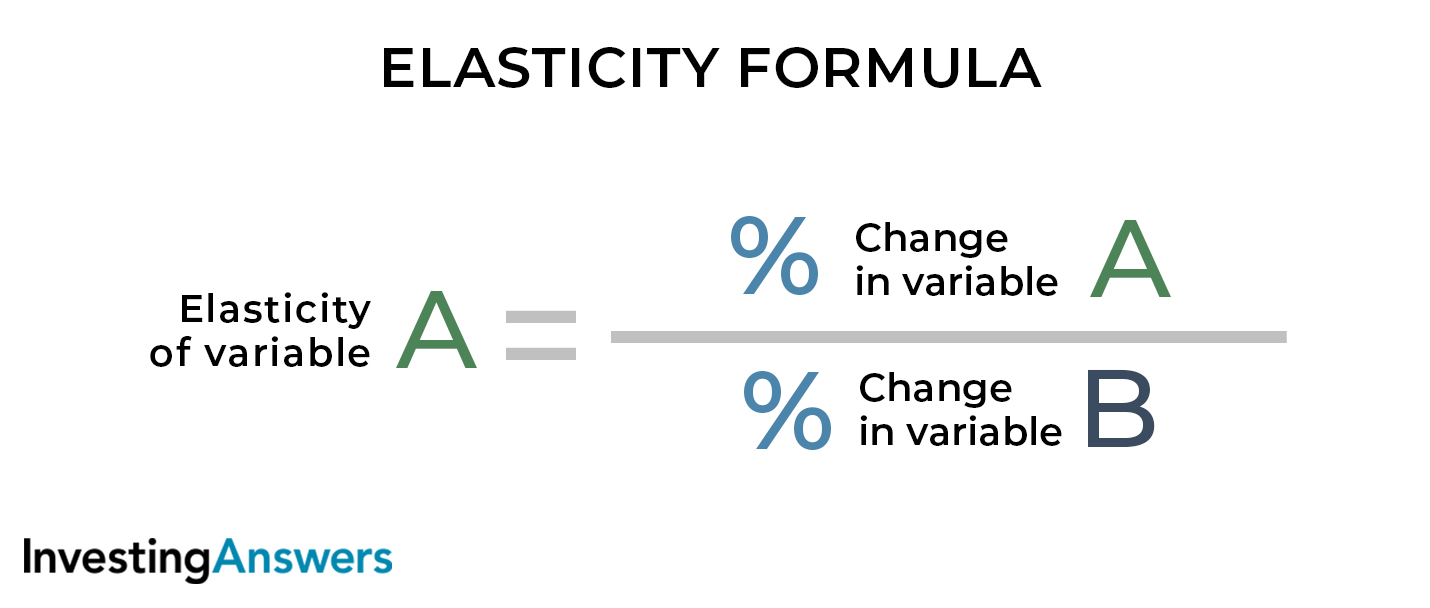## What Does the Value of Elasticity Coefficient Mean?

The value obtained from the above equation is called the elasticity coefficient (which measures the responsiveness of variable A to changes in variable B). The elasticity coefficient can be understood with the following information:

ValueElasticityMeaning
Perfectly elasticAn increase in variable B causes variable A to drop to zero, a decrease in variable B causes variable A to go to infinity
>1Relatively elastic% Change in variable A is greater than the change in variable B
\$1Unitary elastic% Change in variable A is the same as the change in variable B
<1Relatively inelastic% Change in variable A is less than the change in variable B
\$0Perfectly inelasticVariable A is unaffected by changes in variable B

## Types of Elasticity

There are four elasticity types, and each is used to explain the relationship between two economic variables:

• price elasticity of demand

• price elasticity of supply

• cross elasticity of demand

• income elasticity of demand.

### 1. Price Elasticity of Demand (PED)

Price elasticity of demand is a measure of the change in demand for a good in response to a change in its price.

#### PED Formula

The formula for price elasticity of demand is: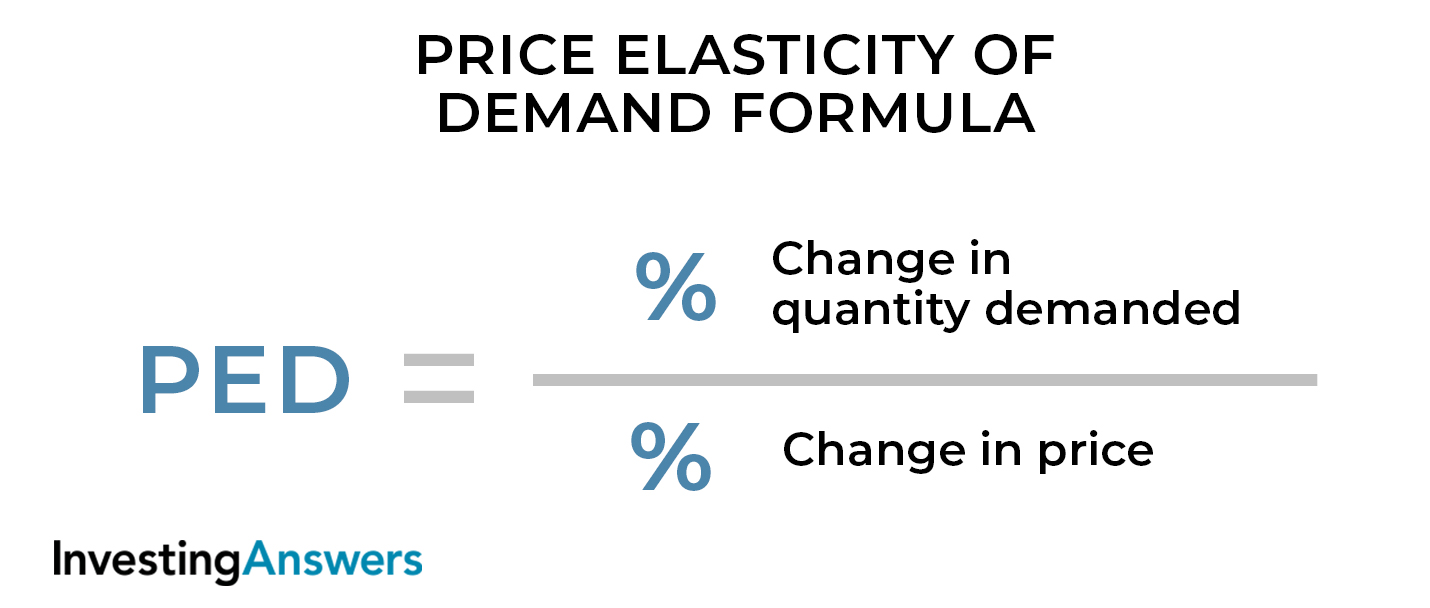### 2. Price Elasticity of Supply (PES)

Price elasticity of supply is a measure of the change in supply of a good in response to a change in its price.

#### PES Formula

The formula for price elasticity of supply is: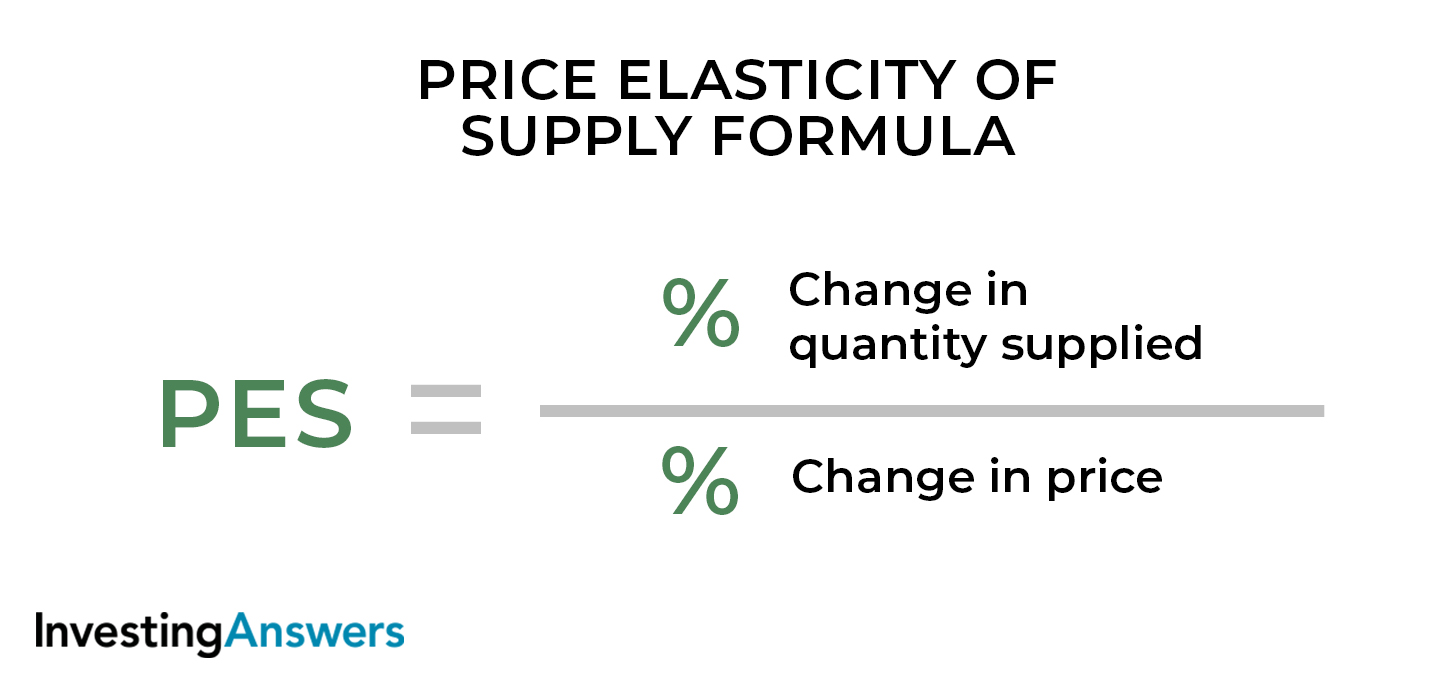### 3. Cross Elasticity of Demand (XED)

Cross elasticity of demand is a measure of the change in demand for a good (in response to a change in the price of another good).

#### XED Formula

The formula for cross elasticity of demand is: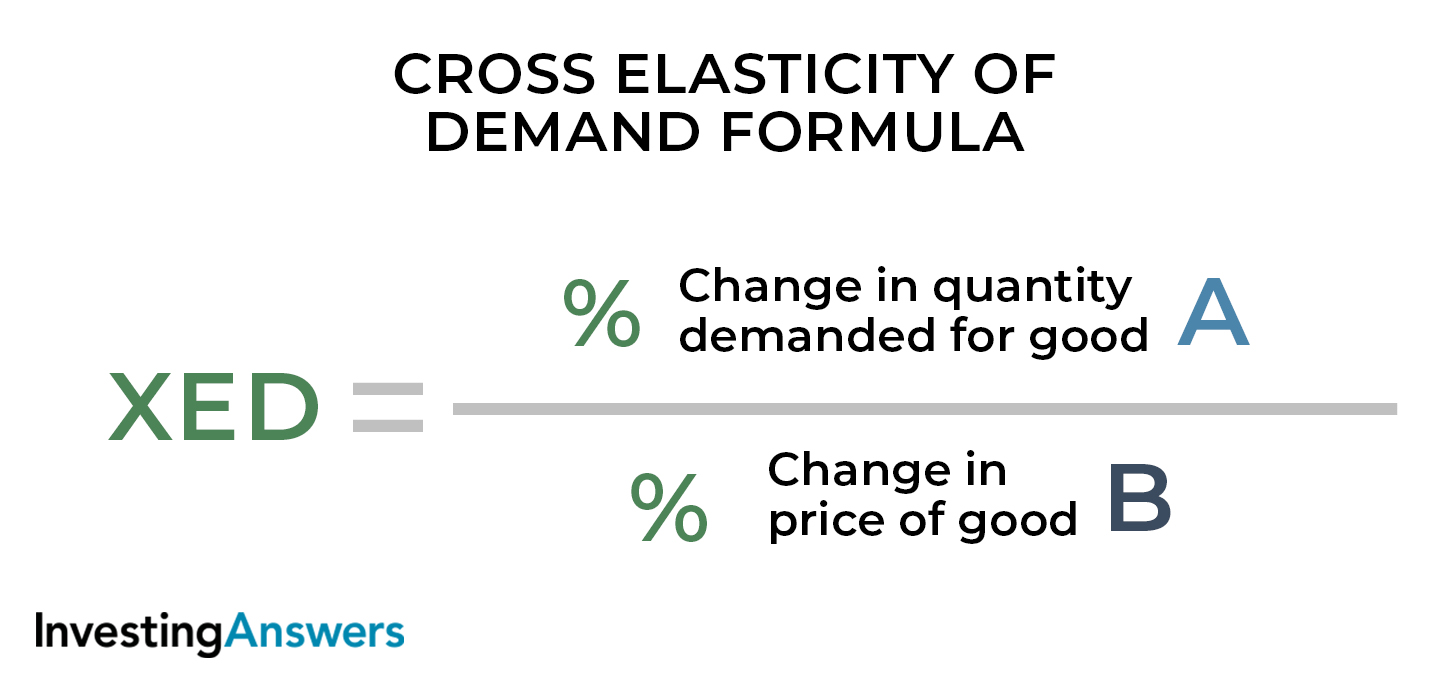### 4. Income Elasticity of Demand (YED)

Income elasticity of demand is a measure of the change in demand for a good (in response to a change in the buyer’s income).

#### YED Formula

The formula for income elasticity of demand is: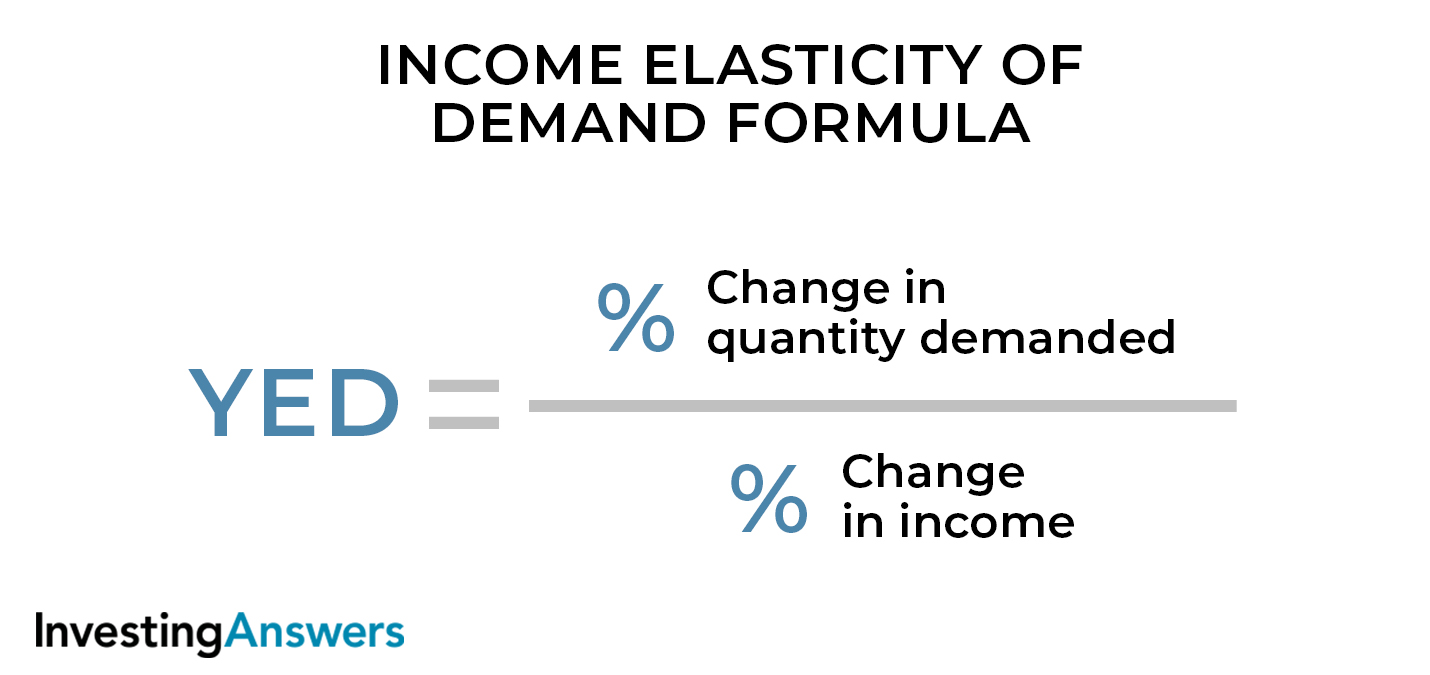All of our content is verified for accuracy by Rachel Siegel, CFA and our team of certified financial experts. We pride ourselves on quality, research, and transparency, and we value your feedback. Below you'll find answers to some of the most common reader questions about Elasticity.

## Is Elasticity Ever Negative?

Yes. In fact, most price elasticity of demand is negative. This reflects the inverse relationship between price and demand for most goods, that is, as price increases (or decreases), the quantity demanded decreases (or increases). For some goods, income elasticity and cross elasticity of demand can also be negative.

## Where Did the Concept of Elasticity Come from?

The first mention of elasticity in economics was published by Alfred Marshall in 1890.

## What Is the Elasticity Coefficient?

The elasticity coefficient is the value found by solving for the elasticity formula. This number tells us whether a variable is perfectly elastic, relatively elastic, unitary elastic, relatively inelastic, or perfectly inelastic.

Rachel Siegel, CFA - 141Rachel Siegel, CFACFA Charterholder

Chartered Financial Analyst

Rachel Siegel, CFA is one of the nation's leading experts at ensuring the accuracy of financial and economic text.  Her prestigious background includes over 10 years creating professional financial certification exams and another 20 years of college-level teaching.